# Unlike Denominators - Definition with Examples

The Complete K-5 Math Learning Program Built for Your Child

• 30 Million Kids

Loved by kids and parent worldwide

• 50,000 Schools

Trusted by teachers across schools

• Comprehensive Curriculum

Aligned to Common Core

What is Unlike Denominators?

Two fractions with non-identical denominators are known as unlike denominators.It is challenging to compare fractions having unlike denominators. Also, performing the operations of addition and subtraction of fractions with unlike denominators is difficult. Hence, to compare and order, or to add and subtract fractions with unlike denominators, we first convert these fractions to like fractions, that is, fractions with same denominators.

For example, to compare the fractions 34 and 57, we first draw the fraction models and then compare the two fractions.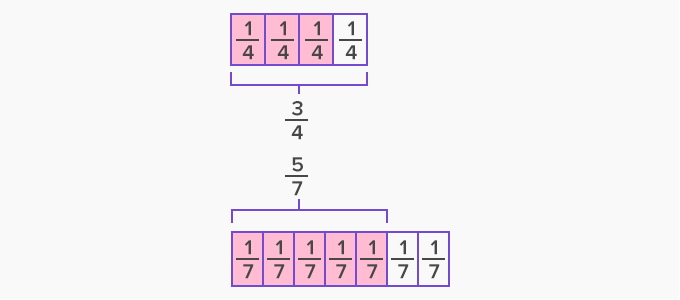Looking at the shaded parts of the figures, we can say that 34 is greater than 5. However, drawing fraction models is not convenient at all times. In such cases, we need to find a way out to compare fractions with unlike denominators.

Converting unlike denominators to like denominators or common denominator:

To convert the fractions with unlike denominators to like denominators or common denominator, we follow the given steps.

Step 1: Find the least common multiple (LCM) of the denominators.

Multiples of 4: 4, 8, 12, 16, 20, 24, 28, 32, 36, 40, ...

Multiples of 7: 7, 14, 21, 28, 35, 42, 49, 56, 63, 70,...

LCM of 4 and 7 is 28

Step 2: Multiply both the numerator and the denominator of each fraction by a number to get the obtained LCM as the denominator.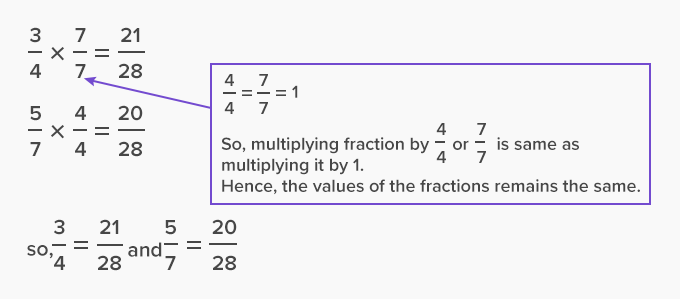Once the fractions have like denominators, they can be easily comapared, added or subtracted.

Example:

 Compare 34 and 57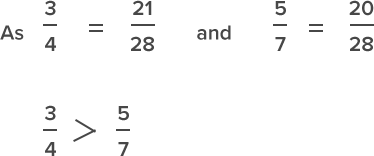Add 34 and57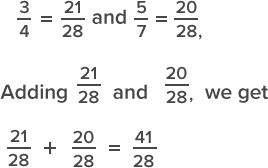Subtract 57 from 34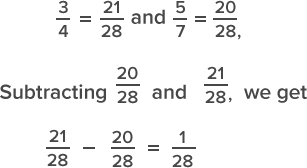Fun Facts Fractions having unlike denominators but like numerators can be compared directly. The fraction with the smaller denominator is always greater.

Example:

49 > 411

Won Numerous Awards & Honors